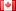# Revision history [back]

### Substituting for a matrix of variables

I constructed a matrix of variables in the following way:

n = 3
R = PolynomialRing(RR,[['r','t'][cmp(int(i/n),i%n)]+'_'+str(1+int(i/n))+str(1+i%n) for i in range(n^2)])
S = matrix(R,3,R.gens())
show(S)

Interestingly though, the following snippet, decidedly the same to me, works with a similar syntax -

xx = [var('alpha_%d'% (i+1)) for i in range(n)] n = 3 R = PolynomialRing(RR,[['r','t'][cmp(int(i/n),i%n)]+'_'+str(1+int(i/n))+str(1+i%n) for i in range(n^2)]) S = matrix(R,3,R.gens()) def kron(i,j): if i==j: return 1 else: return 0 def func(S,xx): return [[(-1/2)*xx[i]*S[i][j]*kron(i,j) for i in range(n)]for j in range(n)] f = matrix(func(S,xx)) diff_S = S*f.conjugate_transpose()*S - f print diff_S({S:0})

Can someone please edit this so as the code markdown shows! I tried, but a left indent of 4 doesnt work for me anymore!

### Substituting for a matrix of variables

I constructed a matrix of variables in the following way:

n = 3
R = PolynomialRing(RR,[['r','t'][cmp(int(i/n),i%n)]+'_'+str(1+int(i/n))+str(1+i%n) for i in range(n^2)])
S = matrix(R,3,R.gens())
show(S)

When i tried to substitute one of the variables with zero, it did not work.
n = 3
R = PolynomialRing(RR,[['r','t'][cmp(int(i/n),i%n)]+'_'+str(1+int(i/n))+str(1+i%n) for i in range(n^2)])
S = matrix(R,3,R.gens())
f = matrix([[S[i][j] for i in range(n)] for j in range(n)])
f({S:0})

Interestingly though, the following snippet, decidedly the same to me, works with a similar syntax -

xx = [var('alpha_%d'% (i+1)) for i in range(n)] n = 3 R = PolynomialRing(RR,[['r','t'][cmp(int(i/n),i%n)]+'_'+str(1+int(i/n))+str(1+i%n) for i in range(n^2)]) S = matrix(R,3,R.gens()) def kron(i,j): if i==j: return 1 else: return 0 def func(S,xx): return [[(-1/2)*xx[i]*S[i][j]*kron(i,j) for i in range(n)]for j in range(n)] f = matrix(func(S,xx)) diff_S = S*f.conjugate_transpose()*S - f print diff_S({S:0})

Can someone please edit this so as the code markdown shows! I tried, but a left indent of 4 doesnt work for me anymore!

### Substituting for a matrix of variables

I constructed a matrix of variables in the following way:

n = 3
R = PolynomialRing(RR,[['r','t'][cmp(int(i/n),i%n)]+'_'+str(1+int(i/n))+str(1+i%n) for i in range(n^2)])
S = matrix(R,3,R.gens())
show(S)


n = 3
R = PolynomialRing(RR,[['r','t'][cmp(int(i/n),i%n)]+'_'+str(1+int(i/n))+str(1+i%n) for i in range(n^2)])
S = matrix(R,3,R.gens())
show(S)

When i tried to substitute one of the variables with zero, it did not work.
work. n = 3
3 R = PolynomialRing(RR,[['r','t'][cmp(int(i/n),i%n)]+'_'+str(1+int(i/n))+str(1+i%n) for i in range(n^2)])
range(n^2)]) S = matrix(R,3,R.gens())
matrix(R,3,R.gens()) f = matrix([[S[i][j] for i in range(n)] for j in range(n)])
f({S:0})
range(n)]) f({S:0})

Interestingly though, the following snippet, decidedly the same to me, works with a similar syntax -

syntax xx = [var('alpha_%d'% (i+1)) for i in range(n)] n = 3 R = PolynomialRing(RR,[['r','t'][cmp(int(i/n),i%n)]+'_'+str(1+int(i/n))+str(1+i%n) for i in range(n^2)]) S = matrix(R,3,R.gens()) def kron(i,j): if i==j: return 1 else: return 0 def func(S,xx): return [[(-1/2)*xx[i]*S[i][j]*kron(i,j) [[(-1/2)xx[i]S[i][j]kron(i,j) for i in range(n)]for j in range(n)] f = matrix(func(S,xx)) diff_S = S*f.conjugate_transpose()*S Sf.conjugate_transpose()*S - f print diff_S({S:0})

Can someone please edit this so as the code markdown shows! I tried, but a left indent of 4 doesnt work for me anymore!4 No.4 RevisionDSM5242 ●21 ●68 ●112

### Substituting for a matrix of variables

I constructed a matrix of variables in the following way:

n = 3
R = PolynomialRing(RR,[['r','t'][cmp(int(i/n),i%n)]+'_'+str(1+int(i/n))+str(1+i%n) for i in range(n^2)])
S = matrix(R,3,R.gens())
show(S)


When i tried to substitute one of the variables with zero, it did not work. work.

n = 3
R = PolynomialRing(RR,[['r','t'][cmp(int(i/n),i%n)]+'_'+str(1+int(i/n))+str(1+i%n) for i in range(n^2)])
S = matrix(R,3,R.gens())
f = matrix([[S[i][j] for i in range(n)] for j in range(n)])
f({S:0})f({S:0})


Interestingly though, the following snippet, decidedly the same to me, works with a similar syntax syntax

xx = [var('alpha_%d'% (i+1)) for i in range(n)]
n = 3
R = PolynomialRing(RR,[['r','t'][cmp(int(i/n),i%n)]+'_'+str(1+int(i/n))+str(1+i%n) for i in range(n^2)])
S = matrix(R,3,R.gens())
def kron(i,j):
if i==j: return 1
else: return 0
def func(S,xx):
return [[(-1/2)xx[i]S[i][j]kron(i,j) [[(-1/2)*xx[i]*S[i][j]*kron(i,j) for i in range(n)]for j in range(n)]
f = matrix(func(S,xx))
diff_S = Sf.conjugate_transpose()*S S*f.conjugate_transpose()*S - f
print diff_S({S:0})diff_S({S:0})